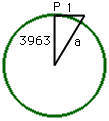Subject: Curvature

Name: RobertHi again Robert, Suppose that the earth is a sphere of radius 3963 miles. If you are at a point P on the earth's surface and move tangent to the surface a distance of 1 mile then you can form a right angled triangel as in the diagram. Using the theorem of Pythagoras a2 = 39632 + 12 = 15705370 and thus a = 3963.000126 miles. Thus your position is 3963.000126 - 3963 = 0.000126 miles above the surface of the earth. 0.000126 miles = 12*5280*0.000126 = 7.98 inches. Hence the earth's surface curves approximately 8 inches in one mile. Look at our response to Shirley to see what happens after the first mile. Cheers, Harley